# Trees with Minimum Weighted Path Length:Introduction and Huffman Trees.

###### Introduction

The concept of the “weighted path length” is important in data compression and searching. In case of data compression lengths of paths correspond to lengths of code-words. In case of searching they correspond to the number of elementary searching steps. By a length of a path we mean usually its number of edges.

Assume we have n weighted items, where wi is the non-negative weight of the ith item.

We denote the sequence of weights by S = (w1 ... wn ). We adopt the convention that the items have unique names. When convenient to do so, we will assume that those names are the positions of items in the list, namely integers in [1 ... n].

We consider a binary tree T , where the items are placed in vertices of the trees (in leaves only or in every node, depending on the speciﬁc problem). We deﬁne the minimum weighted path length (cost) of the tree T as follows: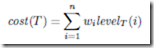where level T is the level function of T , i.e., level T (i) is the level (or depth) of i in T , deﬁned to be the length of the path in T from the root to i.

In some special cases (lopsided trees) the edges can have diﬀerent lengths and the path length in this case is the sum of individual lengths of edges on the path.

In this chapter we concentrate on several interesting algorithms in the area:

• Huﬀman algorithm constructing optimal preﬁx-free codes in time O(n log n), in this case the items are placed in leaves of the tree, the original order of items can be diﬀerent from their order as leaves;

• A version of Huﬀman algorithm which works in O(n) time if the weights of items are sorted

• Larmore-Hirschberg algorithm for optimal height-limited Huﬀman trees working in time O(n × L), where L is the upper bound on the height, it is an interesting algorithm transforming the problem to so called “coin-collector”, see .

• Construction of optimal binary search trees (OBST) in O(n2) time using certain property of monotonicity of “splitting points” of subtrees. In case of OBST every node (also internal) contains exactly one item. (Binary search trees are deﬁned in Chapter 3.)

• Construction of optimal alphabetic trees (OAT) in O(n log n) time: the Garsia-Wachs algorithm . It is a version of an earlier algorithm of Hu-Tucker [12, 18] for this problem. The correctness of this algorithm is nontrivial and this algorithm (as well as Hu-Tucker) and these are the most interesting algorithms in the area.

• Construction of optimal lopsided trees, these are the trees similar to Huﬀman trees except that the edges can have some lengths speciﬁed.

• Short discussion of parallel algorithms Many of these algorithms look “mysterious”, in particular the Garsia-Wachs algorithm for optimal alphabetic trees. This is the version of the Hu-Tucker algorithm. Both algorithms are rather simple to understand in how they work and their complexity, but correctness is a complicated issue.

Similarly one can observe a mysterious behavior of the Larmore-Hirschberg algorithm for height-limited Huﬀman trees. Its “mysterious” behavior is due to the strange reduction to the seemingly unrelated problem of the coin collector.

The algorithms relating the cost of binary trees to shortest paths in certain graphs are also not intuitive, for example the algorithm for lopsided trees, see , and parallel algorithm for alphabetic trees, see . The eﬃciency of these algorithms relies on the Monge property of related matrices. Both sequential and parallel algorithms for Monge matrices are complicated and interesting.

The area of weighted paths in trees is especially interesting due to its applications (compression, searching) as well as to their relation to many other interesting problems in com- binatorial algorithmics.

###### Huﬀman Trees

Assume we have a text x of length N consisting of n diﬀerent letters with repetitions. The alphabet is a ﬁnite set Σ. Usually we identify the i-th letter with its number i. The letter i appears wi times in x. We need to encode each letter in binary, as h(a), where h is a morphism of alphabet Σ into binary words, in a way to minimize the total length of encoding and guarantee that it is uniquely decipherable, this means that the extension of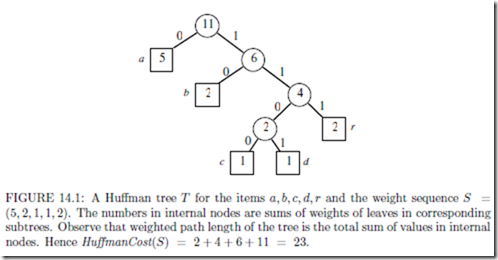the morphism h to all words over Σ is one-to-one. The words h(a), where a Σ, are called codewords or codes.

The special case of uniquely decipherable codes are preﬁx-free codes: none of the code is a preﬁx of another one. The preﬁx-free code can be represented as a binary tree, with left edges corresponding to zeros, and right edge corresponding to ones.

Let S = {w1, w2,…, wn} be the sequence of weights of items. Denote by Huﬀman Cost(S) the total cost of minimal encoding (weighted sum of lengths of code-words) and by HT(S) the tree representing an optimal encoding. Observe that several diﬀerent optimal trees are possible. The basic algorithm is a greedy algorithm designed by Huﬀman, the corresponding trees and codes are called Huﬀman trees and Huﬀman codes.

Example Let text = abracadabra. The number of occurrences of letters are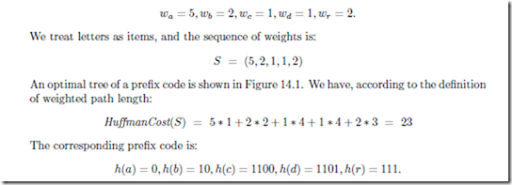We can encode the original text abracadabra using the codes given by paths in the preﬁx tree. The coded text is then 01011101100011010101110, that is a word of length 23.

If for example the initial code words of letters have length 5, we get the compression ratio 55/23 2.4.

O(n log n) Time Algorithm

The basic algorithm is the greedy algorithm given by Huﬀman. In this algorithm we can assume that two items of smallest weight are at the bottom of the tree and they are sons of a same node. The greedy approach in this case is to minimize the cost locally.

Two smallest weight items are combined into a single package with weight equal to the sum of weights of these small items. Then we proceed recursively. The following observation is used.

Observation Assume that the numbers in internal nodes are sums of weights of leaves in corresponding subtrees. Then the total weighted path length of the tree is the total sum of values in internal nodes.where u, w are two minimal elements of S. This implies the following algorithm, in which we assume that initially S is stored in a min-priority queue. The algorithm is presented below as a recursive function HuﬀmanCost(S) but it can be easily written without recursion. The algorithm computes only the total cost.

THEOREM 14.1 Huﬀman algorithm constructs optimal tree in O(n log n) time

Proof In an optimal tree we can exchange two items which are sons of a same father at a bottom level with two smallest weight items. This will not increase the cost of the tree. Hence there is an optimal tree with two smallest weight items being sons of a same node. This implies correctness of the algorithm.

The complexity is O(n log n) since each operation in the priority queue takes O(log n) time and there are O(n) operations of Extract-Min.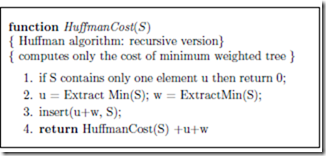The algorithm in fact computes only the cost of Huﬀman tree, but the tree can be created on-line in the algorithm. Each time we combine two items we create their father and create links son-father. In the course of the algorithm we keep a forest (collection of trees). Eventually this becomes a single Huﬀman tree at the end.

Linear Time Algorithm for Presorted Sequence of Items

There is possible an algorithm using “simple” queues with constant time operations of inserting and deleting elements from the queue if the items are presorted.

THEOREM 14.2 If the weights are already sorted then the Huﬀman tree can be con- structed in linear time.

Proof If we have sorted queues of remaining original items and remaining newly created items (packages) then it is easy to see that two smallest items can be chosen from among 4 items, two ﬁrst elements of each queue. This proves correctness.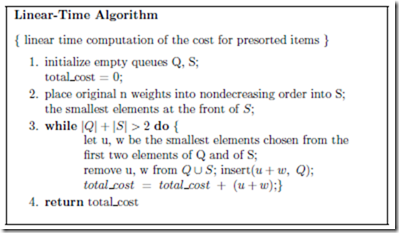Relation between General Uniquely Decipherable Codes and Preﬁx-free Codes

It would seem that, for some weight sequences, in the class of uniquely decipherable codes there are possible codes which beat every Huﬀman (preﬁx-free) code. However it happens that preﬁx-free codes are optimal within the whole class of uniquely decipherable codes. It follows immediately from the next three lemmas.

LEMMA 14.1 For each full (each internal node having exactly two sons) binary tree T with leaves 1 ... n we have: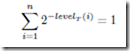Proof Simple induction on n.

LEMMA 14.2 For each uniquely decipherable code S with word lengths £1, £2,... , £k on the alphabet {0, 1} we have :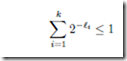Proof For a set W of words on the alphabet {0, 1} deﬁne: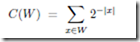We have to show that C(S) 1. Let us ﬁrst observe the following simple fact.

Observation.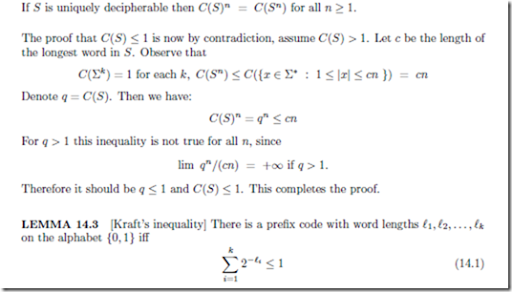Proof It is enough to show how to construct such a code if the inequality holds. Assume the lengths are sorted in the increasing order. Assume we have a (potentially) inﬁnite full binary tree. We construct the next codeword by going top-down, starting from the root. We assign to the i-th codeword, for i = 1, 2, 3,... , k, the lexicographically ﬁrst path of length £i, such that the bottom node of this path has not been visited before. It is illustrated in Figure 14.2. If the path does not exist then this means that in this moment we covered with paths a full binary tree, and the actual sum equals 1. But some other lengths remained, so it would be :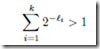a contradiction. This proves that the construction of a preﬁx code works, so the corre- sponding preﬁx-free code covering all lengths exists. This completes the proof.

The lemmas imply directly the following theorem.

THEOREM 14.3 A uniquely decipherable code with prescribed word lengths exists iﬀ a preﬁx code with the same word lengths exists.

We remark that the problem of testing unique decipherability of a set of codewords is complete in nondeterministic logarithmic space, see .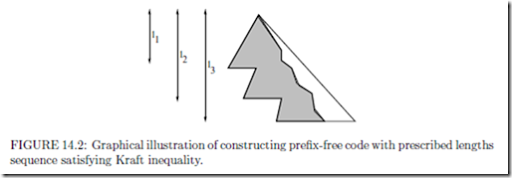Huﬀman Codes and Entropy

The performance of Huﬀman codes is related to a measure of information of the source text, called the entropy (denoted by E) of the alphabet. Let wa be na/N , where na is the number

of occurrences of a in a given text of length N . In this case the sequence S of weights wa The quantity wa can be now viewed as the probability that letter a occurs at a given position in the text. This probability is assumed to be independent of the position. Then, the entropy of the alphabet according to wa’s is deﬁned as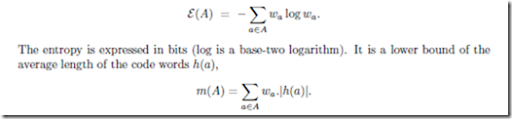Moreover, Huﬀman codes give the best possible approximation of the entropy (among methods based on coding of the alphabet). This is summarized in the following theorem whose proof relies on the inequalities from Lemma 14.2.

THEOREM 14.4 Assume the weight sequence A of weights is normalized. The total cost m(A) of any uniquely decipherable code for A is at least E(A), and we have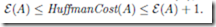Huﬀman Algorithm for t-ary Trees

An important generalization of Huﬀman algorithm is to t-ary trees. Huﬀman algorithm generalizes to the construction of preﬁx codes on alphabet of size t > 2. The trie of the code is then an almost full t-ary tree.

We say that t-ary tree is almost full if all internal nodes have exactly t sons, except possibly one node, which has less sons, in these case all of them should be leaves (let us call this one node a defect node).

We perform similar algorithm to Huﬀman method for binary trees, except that each time we select t items (original or combined) of smallest weight.

There is one technical diﬃculty. Possibly we start by selecting a smaller number of items in the ﬁrst step. If we know t and the number of items then it is easy to calculate number q of sons of the defect node, for example if t = 3 and n = 8 then the defect node has two sons. It is easy to compute the number q of sons of the defect node due to the following simple fact.

LEMMA 14.4 If T is a full t-ary tree with m leaves then m modulo (t 1) = 1.

We start the algorithm by combining q smallest items. Later each time we combine exactly t values. To simplify the algorithm we can add the smallest possible number of dummy items of weigh zero to make the tree full t-ary tree.